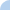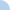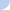Algebra 2H: sequence quiz #1

For each of the following, you will be given a sequence of numbers. Determine if the sequence is arithmetic, geometric, neither, or both. You will only have TWO opportunities to take this quiz. There are 6 questions.

 This quiz is timed. The total time allowed for this quiz is 1 hour.Quiz Log In